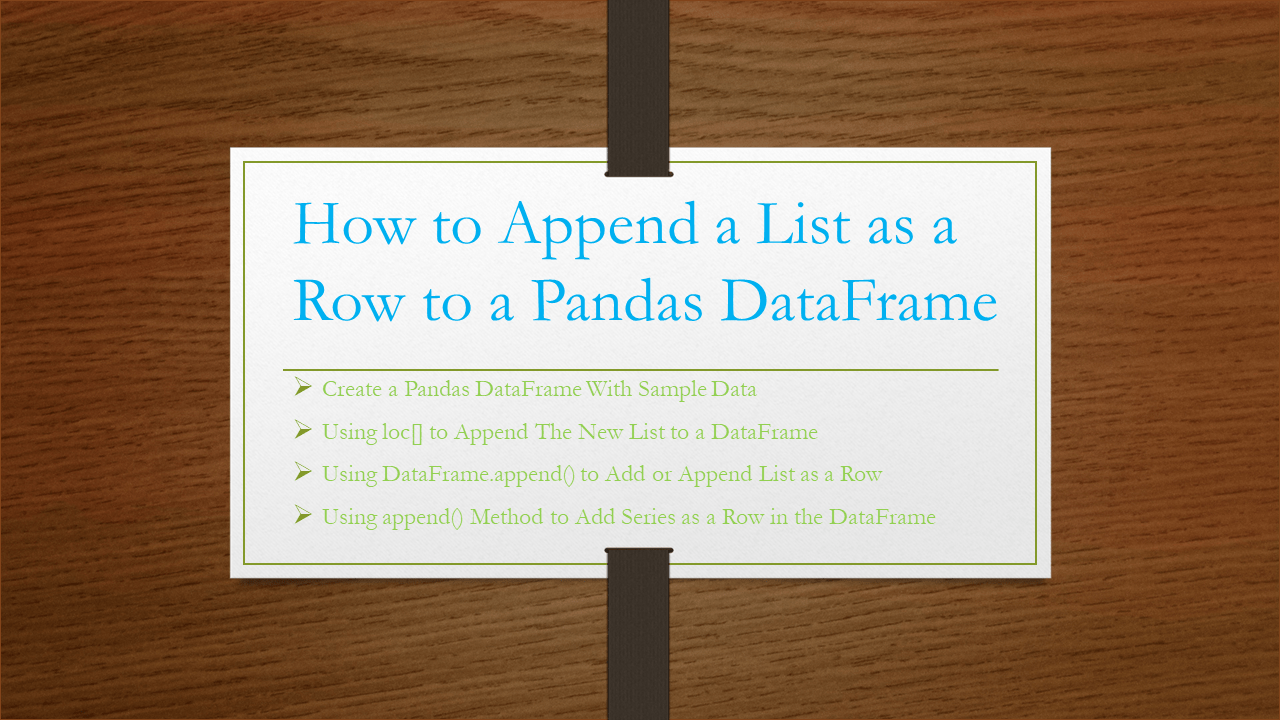# Pandas – Append a List as a Row to DataFrame

pandas support several ways to append a list as a row to DataFrame, In this article, I will explain how to append a Python list as a row where it adds a new row to the DataFrame with elements specified by a list.

You can use the append() method, loc[], and iloc[] properties to append/add a list of values as a row in Pandas, let’s see these with examples. Use loc vs iloc to understand the differences.

## 1. Quick Examples of pandas append list to DataFrame

Below are some quick examples of appending a list as a row to DataFrame.

``````
# Below are some quick examples

# New list to append Row to DataFrame
list = ["Hyperion", 27000, "60days", 2000]
df.loc[len(df)] = list

df.iloc = list

# Using append()
list = ["Bigdata", 27000, "40days", 2800]
df2 = df.append(pd.DataFrame([list],
columns=["Courses","Fee","Duration","Discount"]),
ignore_index=True)

# A series object with the same index as DataFrame
df2 = df.append(pd.Series(list, index = ["Courses","Fee","Duration","Discount"]),
ignore_index=True)
``````

Let’s create a DataFrame with a few rows and columns then, execute some examples and validate the results. Our DataFrame contains column names `Courses`, `Fee`, `Duration` and `Discount`.

``````
# Create DataFrame
import pandas as pd
technologies= {
'Fee' :[22000,25000,23000,24000,26000],
'Duration':['30days','50days','35days', '40days','55days'],
'Discount':[1000,2300,1000,1200,2500]
}
df = pd.DataFrame(technologies)
print(" Create DataFrame:\n", df)
``````

Yields below output.

## 2. Using loc[] to Append The New List to a DataFrame

By using `df.loc[index]=list` you can append a list as a row to the DataFrame at a specified Index, In order to add at the end get the index of the last record using `len(df)` function. The below example adds the list `["Hyperion",27000,"60days",2000]` to the end of the Pandas DataFrame.

``````
# New list to append Row to DataFrame
list = ["Hyperion", 27000, "60days", 2000]
df.loc[len(df)] = list
print("After appending a row as a list:\n", df)
``````

Yields below output.

Use `df.iloc=list` to append the row to the second position of the DataFrame as the Index starts from zero.

``````
# New list to append DataFrame
list = ["Oracle", 20000, "60days", 2000]
# using iloc[] method
df.iloc = list
print(df)
``````

Yields below output.

``````
# Output:
Courses    Fee Duration  Discount
0   Spark  22000   30days      1000
1  Oracle  20000   60days      2000
3  Python  24000   40days      1200
4  Pandas  26000   55days      2500
``````

## 3. Using DataFrame.append() to Add or Append a List as a Row

Alternatively, you can use DataFrame.append() function to add a row to the DataFrame. This by default adds a row at the end. You can Get Column Names as a List From Pandas DataFrame and use it in this example.

``````
# New list for append into DataFrame
list = ["Bigdata", 27000, "40days", 2800]

# Using append to add the list to DataFrame
df2 = df.append(pd.DataFrame([list], columns=["Courses","Fee","Duration","Discount"]), ignore_index=True)
print(df2)
``````

Yields below output.

``````
# Output:
Courses    Fee Duration  Discount
0    Spark  22000   30days      1000
1  PySpark  25000   50days      2300
3   Python  24000   40days      1200
4   Pandas  26000   55days      2500
5  Bigdata  27000   40days      2800
``````

## 4. Append Series as a Row to the DataFrame

Using append() you can also append series as a row to the DataFrame.

``````
# New list for append into DataFrame
list = ["Bigdata", 27000, "40days", 2800]

# A series object with the same index as DataFrame
df2 = df.append(pd.Series(list, index = ["Courses","Fee","Duration","Discount"]), ignore_index=True)
print(df2)
``````

Yields below output.

``````
# Output:
Courses    Fee Duration  Discount
0    Spark  22000   30days      1000
1  PySpark  25000   50days      2300
3   Python  24000   40days      1200
4   Pandas  26000   55days      2500
5  Bigdata  27000   40days      2800
``````

## Conclusion

In this article, you have learned how to append a list as a row to Pandas DataFrame using `DataFrame.loc[]`, `DataFrame.iloc[]`, `DataFrame.append()` methods. Using these you can append a row at any position/index.

Happy Learning !!

### Naveen (NNK)

Naveen (NNK) is a Data Engineer with 20+ years of experience in transforming data into actionable insights. Over the years, He has honed his expertise in designing, implementing, and maintaining data pipelines with frameworks like Apache Spark, PySpark, Pandas, R, Hive and Machine Learning. Naveen journey in the field of data engineering has been a continuous learning, innovation, and a strong commitment to data integrity. In this blog, he shares his experiences with the data as he come across. Follow Naveen @ @ LinkedIn

### This Post Has 2 Comments

1.Anonymous

.append for pd dfs is depreciated

2.Anonymous

.append for pd dfs is depreciated